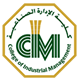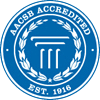#Dr. Amine Nehari Talet

Associate Professor,

Department of Accounting & MIS

We never miss the water till the well runs dry

Formula for Success

A small truth to make our Life's 100% successful..........

If A B C D E F G H I J K L M N O P Q R S T U V W X Y Z

Is equal to 1 2 3 4 5 6 7 8 9 10 11 12 13 14 15 16 17 18 19 20 21 22 23 24 25 26

Then H+A+R+D+W+O+R+K =

8+1+18+4+23+15+18+11 = 98%

K+N+O+W+L+E+D+G+E = 11+14+15+23+12+5+4+7+5 = 96%

L+U+C+K = 12+21+3+11 = 47%

(None of them makes 100%)  Then what makes 100%

Is it Money? ..... No!!!!!    Leadership? ...... NO!!!!

Every problem has a solution, only if we perhaps change our "ATTITUDE".

It is OUR  ATTITUDE towards Life and Work that makes OUR Life 100% Successful...

A+T+T+I+T+U+D+E= 1+20+20+9+20+21+4+5 = 100%